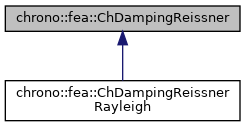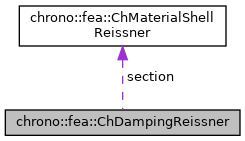chrono::fea::ChDampingReissner Class Referenceabstract

## Description

Base interface for damping of 6-field Reissner-Mindlin shells (kinematically-exact shell theory as in Witkowski et al.) to be used in a ChMaterialShellReissner.

Children classes should implement a ComputeStress function that returns generalized stresses given time derivatives of strains as: {n_u,n_v,m_u.m_v}=f({e_u',e_v',k_u',k_v'})

#include <ChMaterialShellReissner.h>

Inheritance diagram for chrono::fea::ChDampingReissner:[legend]
Collaboration diagram for chrono::fea::ChDampingReissner:[legend]

## Public Member Functions

virtual void ComputeStress (ChVector<> &n_u, ChVector<> &n_v, ChVector<> &m_u, ChVector<> &m_v, const ChVector<> &deps_u, const ChVector<> &deps_v, const ChVector<> &dkur_u, const ChVector<> &dkur_v, const double z_inf, const double z_sup, const double angle)=0
Compute the generalized cut force and cut torque, caused by structural damping, given actual deformation speed and curvature speed. More...

virtual void ComputeDampingMatrix (ChMatrixRef R, const ChVector<> &deps_u, const ChVector<> &deps_v, const ChVector<> &dkur_u, const ChVector<> &dkur_v, const double z_inf, const double z_sup, const double angle)
Compute the 12x12 tangent material damping matrix, ie the jacobian [Rm]=dstress/dstrainspeed. More...

## Public Attributes

ChMaterialShellReissnersection

## ◆ ComputeDampingMatrix()

 void chrono::fea::ChDampingReissner::ComputeDampingMatrix ( ChMatrixRef R, const ChVector<> & deps_u, const ChVector<> & deps_v, const ChVector<> & dkur_u, const ChVector<> & dkur_v, const double z_inf, const double z_sup, const double angle )
virtual

Compute the 12x12 tangent material damping matrix, ie the jacobian [Rm]=dstress/dstrainspeed.

This must be overridden by subclasses if an analytical solution is known (preferred for high performance), otherwise the base behaviour here is to compute [Rm] by numerical differentiation calling ComputeStress() multiple times.

Parameters
 R 12x12 material damping matrix values here deps_u time derivative of strains along u direction deps_v time derivative of strains along v direction dkur_u time derivative of curvature along u direction dkur_v time derivative of curvature along v direction z_inf layer lower z value (along thickness coord) z_sup layer upper z value (along thickness coord) angle layer angle respect to x (if needed) -not used in this, isotropic

Reimplemented in chrono::fea::ChDampingReissnerRayleigh.

## ◆ ComputeStress()

 virtual void chrono::fea::ChDampingReissner::ComputeStress ( ChVector<> & n_u, ChVector<> & n_v, ChVector<> & m_u, ChVector<> & m_v, const ChVector<> & deps_u, const ChVector<> & deps_v, const ChVector<> & dkur_u, const ChVector<> & dkur_v, const double z_inf, const double z_sup, const double angle )
pure virtual

Compute the generalized cut force and cut torque, caused by structural damping, given actual deformation speed and curvature speed.

This MUST be implemented by subclasses.

Parameters
 n_u forces along u direction (per unit length) n_v forces along v direction (per unit length) m_u torques along u direction (per unit length) m_v torques along v direction (per unit length) deps_u time derivative of strains along u direction deps_v time derivative of strains along v direction dkur_u time derivative of curvature along u direction dkur_v time derivative of curvature along v direction z_inf layer lower z value (along thickness coord) z_sup layer upper z value (along thickness coord) angle layer angle respect to x (if needed)

Implemented in chrono::fea::ChDampingReissnerRayleigh.

The documentation for this class was generated from the following files:
• /builds/uwsbel/chrono/src/chrono/fea/ChMaterialShellReissner.h
• /builds/uwsbel/chrono/src/chrono/fea/ChMaterialShellReissner.cpp